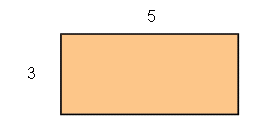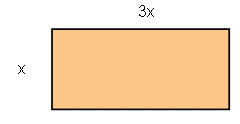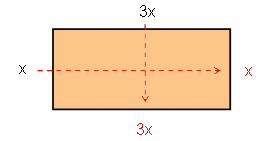SEARCH HOMEMath Central Quandaries & QueriesQuestion from Amy, a student: A rectangle has sides of 3x-4 and 7x+10. Find the expression that represents its perimeter.We have two responses for you.

Hi Amy.

The perimeter of any rectangle is the sum of the lengths of its sides. A rectangle has two pairs of equal length sides, so its perimeter is width + width + length + length, or in other words twice the sum of the width and the length.

The width and the length of your rectangle are 3x-4 and 7x+10, so twice the width plus the length is...?

Stephen La Rocque.>

Hi Amy,

Consider the following rectangle:The perimeter for this one isn't too hard - you just write down the sum of all the sides. Since we have a rectangle, you know that opposite sides are the same length, so an expression for the perimeter could be written as follows:

P = 3 + 5 + 3 + 5

I started at one of the sides that was 3 units long, and went around the rectangle. I could have also written

P = 23 + 25

Now let's look at an example that uses unknowns:So we have an algebraic expression for the side lengths. But just as before, we know that opposite sides will be equal, so we can fill in the expressions for the other two sides:Now we can getting an expression for the perimeter by writing the sum of the four sides:

P = x + 3x + x + 3x

After we collect like-terms to simplify the expression, we get

P = 8x

Now can you find an expression for the perimeter of your rectangle?

HaleyMath Central is supported by the University of Regina and The Pacific Institute for the Mathematical Sciences.